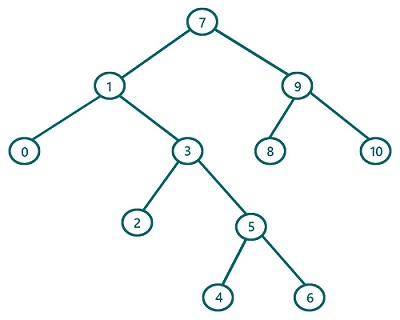# Postorder Traversal in Binary Tree (using recursion) in C, C++

In this article, we are going to find what postorder traversal of a Binary Tree is and how to implement postorder traversal using recursion? We have provided the implementation both in C & C++.
Submitted by Radib Kar, on July 24, 2020

If we classify tree traversals, postorder traversal is one of the traversal techniques which is based on depth-first search traversal.  The basic concept for postorder traversal lies in its name itself. Post means "after" (last/finally) and that's why root is being traversed at last and its subtrees are being traversed first. So, the rule is:

1. First, traverse the left subtree
2. Then traverse the right subtree
3. Finally, traverse the root

Of course, while traversing the subtrees we will follow the same order

So let's traverse the below tree using preorder traversal.For the above tree, the root is: 7

So First Traverse the left subtree (Subtree rooted by 1)

Now to traverse the subtree rooted by 1, it again follows the same set of rules

So the First traverse its left subtree rooted by 0

Since it's a leaf node we can print it as there is no more left subtree. Also, 0 has no right subtree, so traverse 0 and this subtree rooted by 0(which is left subtree of 1) is traversed totally.

Traversal till now: 0

Now, traverse the right subtree of root 1(subtree rooted by 3). It again follows the same rule. After traversal of this subtree, we will have

Traversal till now: 0 2 4 6 5 3

Now finally traverse root 1 as we have completed both its subtrees

Traversal till now: 0 2 4 6 5 3 1

Now we have finished the left subtree traversal of the actual tree (rooted by 7)

Rest is about traversing the right subtree of the root 7 and finally the root itself. So ultimately after the whole traversal, we will have: 0 2 4 6 5 3 1 8 10 9 7

The pseudocode would be:

```void postorder(TreeNode root){
if(root is NULL)
return

//recursively traverse left subtree
postorder (left subtree of root)
// recursively traverse right subtree
postorder (right subtree of root)
//traverse current node
print(root)
}
```

C Implementation:

Tree structure:

```struct tree{
int val;
struct tree* left;
struct tree* right;
};

typedef struct tree TreeNode;
```
```#include <stdio.h>
#include <stdlib.h>

struct tree {
int val;
struct tree* left;
struct tree* right;
};

typedef struct tree TreeNode;

TreeNode* newTree(int data)
{
// Allocate memory for new node
TreeNode* root = (TreeNode*)malloc(sizeof(TreeNode));

// Assign data to this node val
root->val = data;

// Initialize left and right children as NULL
root->left = NULL;
root->right = NULL;
return (root);
}

void postorder(TreeNode* root)
{
//base case
if (root == NULL)
return;

// fisrt traverse left sub tree
postorder(root->left);

//secondly traverse right sub tree
postorder(root->right);

//finally traverse current node
printf("%d ", root->val);
}

int main()
{
//building the tree
TreeNode* t = newTree(7);
t->left = newTree(1);
t->left->left = newTree(0);
t->left->right = newTree(3);
t->left->right->left = newTree(2);
t->left->right->right = newTree(5);
t->left->right->right->left = newTree(4);
t->left->right->right->right = newTree(6);
t->right = newTree(9);
t->right->left = newTree(8);
t->right->right = newTree(10);

printf("postorder traversal of the above tree is:\n");

postorder(t);

return 0;
}
```

Output:

```postorder traversal of the above tree is:
0 2 4 6 5 3 1 8 10 9 7
```

C++ implementation:

```#include <bits/stdc++.h>
using namespace std;

class TreeNode { // tree node is defined
public:
int val;
TreeNode* left;
TreeNode* right;
TreeNode(int data)
{
val = data;
left = NULL;
right = NULL;
}
};

void postorder(TreeNode* root)
{
//base case
if (root == NULL)
return;
// fisrt traverse left sub tree
postorder(root->left);
//secondly traverse right sub tree
postorder(root->right);
//finally traverse current node
printf("%d ", root->val);
}

int main()
{
//building the tree
TreeNode* t = new TreeNode(7);
t->left = new TreeNode(1);
t->left->left = new TreeNode(0);
t->left->right = new TreeNode(3);
t->left->right->left = new TreeNode(2);
t->left->right->right = new TreeNode(5);
t->left->right->right->left = new TreeNode(4);
t->left->right->right->right = new TreeNode(6);
t->right = new TreeNode(9);
t->right->left = new TreeNode(8);
t->right->right = new TreeNode(10);

printf("postorder traversal of the above tree is:\n");

postorder(t);

return 0;
}
```

Output:

```postorder traversal of the above tree is:
0 2 4 6 5 3 1 8 10 9 7
```